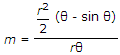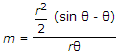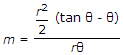# Civil Engineering - Hydraulics

### Exercise :: Hydraulics - Section 3

26.

For pipes not running full, the hydraulic mean depth is given by

 A. m = r(θ - sin θ) B.C.D.Explanation:

No answer description available for this question. Let us discuss.

27.

Atmospheric pressure is equal to water column head of

 A. 9.81 m B. 5.0 m C. 10.30 m D. 7.5 m.

Explanation:

No answer description available for this question. Let us discuss.

28.

Shear stress is directly proportional to

 A. the velocity B. the shear strain C. the viscosity D. the velocity.

Explanation:

No answer description available for this question. Let us discuss.

29.

To avoid an interruption in the flow of a syphon, an air vessel is provided

 A. at the inlet B. at the outlet C. at the summit D. at any point between intet and outlet.

Explanation:

No answer description available for this question. Let us discuss.

30.

Pick up the correct statement from the following :

 A. Total energy gradient is the graphical representation of the total head at any section of a pipe line B. Vertical distance between the total energy line and hydraulic grade line is equal to the velocity head C. Vertical distance between the total energy line and total energy gradient represents loss of head D. all the above.